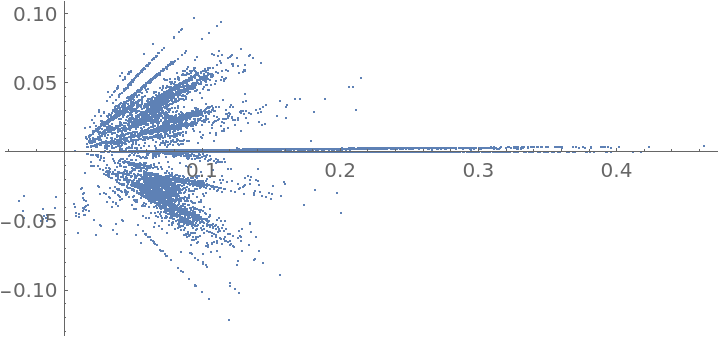#Function Repository Resource:

# GeothmeticMeandian

Calculate the geothmetic meandian of a list of numbers

Contributed by: Sander Huisman
 ResourceFunction["GeothmeticMeandian"][x1,x2,…] gives the geothmetic meandian of the xi. ResourceFunction["GeothmeticMeandian"][list] gives the geothmetic meandian of the elements in list.

## Details

As defined by the xkcd comic website, the geothmetic meandian is the number obtained by iteratively calculating {Mean[list],GeometricMean[list],Median[list]} to convergence.
For negative numbers, the geometric mean generally gives complex numbers. For complex numbers, the Median is modified so as to order by the absolute value of the numbers.

## Examples

### Basic Examples (1)

Compute the geothmetic meandian of some numbers:

 In:=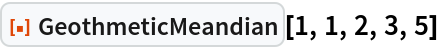Out=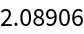### Scope (3)

The input can be given as multiple arguments:

 In:=Out=The input can also be given as a list:

 In:=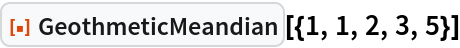Out=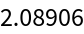The input can also contain complex numbers:

 In:=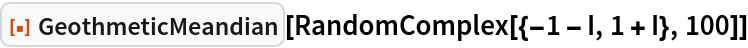Out=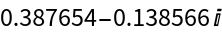### Possible Issues (2)

Due to the GeometricMean operation, negative numbers can result in complex answers:

 In:=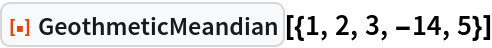Out=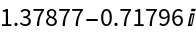Sometimes a real result is returned:

 In:=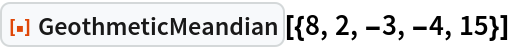Out=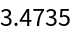Symbolic values are not allowed and will be left unevaluated:

 In:=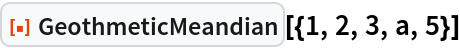Out=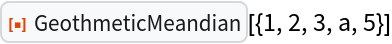### Neat Examples (1)

Find the distribution of the geothmetic meandian of 100 random variates of a normal distribution:

 In:=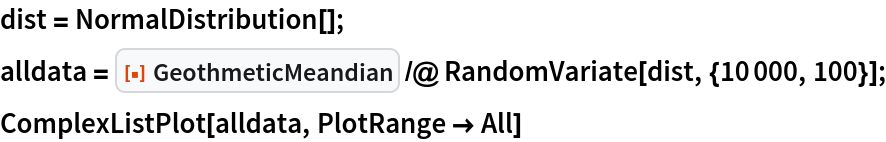Out=